Test: Introduction To Heron's Formula

# Test: Introduction To Heron's Formula

Test Description

## 20 Questions MCQ Test Mathematics (Maths) Class 9 | Test: Introduction To Heron's Formula

Test: Introduction To Heron's Formula for Class 9 2023 is part of Mathematics (Maths) Class 9 preparation. The Test: Introduction To Heron's Formula questions and answers have been prepared according to the Class 9 exam syllabus.The Test: Introduction To Heron's Formula MCQs are made for Class 9 2023 Exam. Find important definitions, questions, notes, meanings, examples, exercises, MCQs and online tests for Test: Introduction To Heron's Formula below.
Solutions of Test: Introduction To Heron's Formula questions in English are available as part of our Mathematics (Maths) Class 9 for Class 9 & Test: Introduction To Heron's Formula solutions in Hindi for Mathematics (Maths) Class 9 course. Download more important topics, notes, lectures and mock test series for Class 9 Exam by signing up for free. Attempt Test: Introduction To Heron's Formula | 20 questions in 20 minutes | Mock test for Class 9 preparation | Free important questions MCQ to study Mathematics (Maths) Class 9 for Class 9 Exam | Download free PDF with solutions
 1 Crore+ students have signed up on EduRev. Have you?
Test: Introduction To Heron's Formula - Question 1

### The area of a triangle is 150 cm2 and its sides are in the ratio 3 : 4 : 5. What is its perimeter?

Detailed Solution for Test: Introduction To Heron's Formula - Question 1

Let coefficient of ratios be X
a = 3x
b = 4x
c = 5x

Using Heron's Formula,
s = (3+4+5)x/2 = 12x/2 = 6x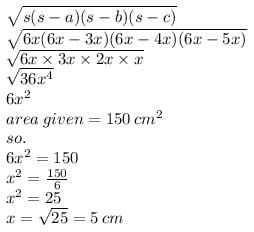Now,
a = 5*3 = 15 cm
b = 5*4 = 20 cm
c = 5*5 = 25 cm

Perimeter = 15+20+25 = 60 cm

Test: Introduction To Heron's Formula - Question 2

### The area of triangle, whose sides are 15 cm, 25 cm and 14 cm:

Detailed Solution for Test: Introduction To Heron's Formula - Question 2

Let
a = 15cm
b = 25cm
c = 14cm

Semi-Perimeter = a + b + c / 2
= 15 + 25 + 14 / 2
= 54 / 2
= 27 cm.

Area Through Heron's Formula

= √s(s-a)(s-b)(s-c)
= √27(27-15)(27-25)(27-14)
= √27 × 12 × 2 × 13
= √3 × 3 × 3 × 2 × 2 × 3 × 13
= 3 × 3 × 2 √13
= 18 √13

Test: Introduction To Heron's Formula - Question 3

### The area of an equilateral triangle of side 6 cm is​

Test: Introduction To Heron's Formula - Question 4

The perimeter of a rhombus is 146 cm. One of its diagonals is 55 cm. The length of the other diagonal and area of the rhombus are​

Detailed Solution for Test: Introduction To Heron's Formula - Question 4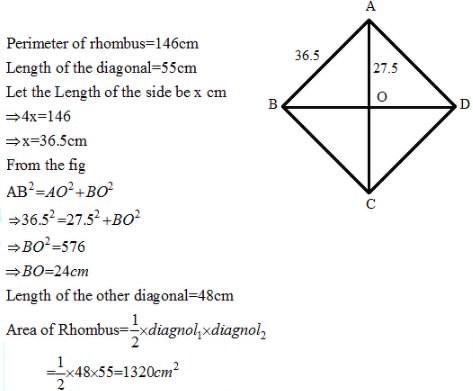Test: Introduction To Heron's Formula - Question 5

The sides of a triangle are in the ratio of 3 : 4 : 5. If its perimeter is 36 cm, then what is its area?

Detailed Solution for Test: Introduction To Heron's Formula - Question 5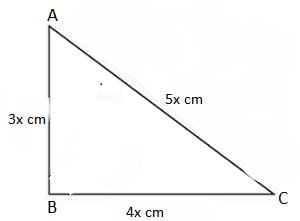⇒ AB + BC + CA = 36 cm

⇒ 3x + 4x + 5x = 36 cm

⇒ 12x = 36

⇒ x = 3

∴ AB = 3x = 9

BC = 4x = 12

CA = 5x = 15

Now, (AB)2 + (BC)2

=  (9)2 + (12)2

=  81 + 144

=  255

= (AC)2

Δ ABC is a right angle triangle and ∠B is right angle.

Area of Δ ABC = 1/2 (AB x BC)

= 1/2 (9 x12)

= 54 cm2

Test: Introduction To Heron's Formula - Question 6

If each side of an equilateral triangle is increased by 2 cm, then its area increases by 3√3 cm2. The length of its each side and its area are respectively equal to

Test: Introduction To Heron's Formula - Question 7

The area of quadrilateral PQRS, in which PQ = 7 cm, QR = 6 cm, RS = 12 cm, PS = 15 cm and PR = 9 cm:​

Detailed Solution for Test: Introduction To Heron's Formula - Question 7

s = a + b + c /2 = 12 + 9 + 15 /2 = 18cm

Area of PRS = √s(s - a)(s - b)(s - c) = 54 cm^2

s = a + b + c /2 = 7 + 6 + 9 /2 = 11 cm

Area of RPQ = √s(s - a)(s - b)(s - c) = 20.98 cm^2

Area of Quadrilateral = Area of RPQ + Area of PRS

= 54 + 20.98

= 74.98 cm^2

Test: Introduction To Heron's Formula - Question 8

The base of an isosceles triangle is 10 cm and one of its equal sides is 13 cm. The area of the triangle is

Test: Introduction To Heron's Formula - Question 9

The lengths of a triangle are 6 cm, 8 cm and 10 cm. Then the length of perpendicular from the opposite vertex to the side whose length is 8cm is:

Test: Introduction To Heron's Formula - Question 10

The perimeter of an isosceles right angled triangle having an area of 200 cm2 is

Detailed Solution for Test: Introduction To Heron's Formula - Question 10

Area of isosceles right angled triangle =  1/2 *b*h

Here if it is isosceles right angled triangle ,

Then perpendicular = base = x

So, area =1/2 *x*x

400 = x²

x² = 400

x =  = 20 cm

so   P = B = 20

By Pythagoras theorem ,

H² = P² +B²

= (20)²+(20)²

= 400 + 400

= 800

H = 20 root 2 cm

Perimeter = 20 + 20 + 20 root 2

= 40+20 root 2

= 68.28 cm

Test: Introduction To Heron's Formula - Question 11

A carpenter had to make a triangle with sides 5, 6, 5 units. By mistake he made one with sides 5, 8, 5 units. The difference between their areas is:

Detailed Solution for Test: Introduction To Heron's Formula - Question 11

area of actual triangle that he had to make

s = (5+5+6)/2

s= 8

a = √(8 x 3 x 3 x 2)

a1= 12 sq units

area of triangle that the carpenter made

s = (5+5+8)/2

s = 9

a = √(9 x 4 x 4 x 1)

a2 = 12 sq units

so difference a1 - a2 = 12 - 12 =0 sq units

Test: Introduction To Heron's Formula - Question 12

The area of a right triangle of height 15 m and base 20 m is​

Test: Introduction To Heron's Formula - Question 13

The sides of a triangle are in the ratio of 3 : 4 : 5. If its perimeter is 36 cm, then what is its area?

Test: Introduction To Heron's Formula - Question 14

The area of an equilateral triangle of side 14 cm is

Test: Introduction To Heron's Formula - Question 15

The sides of a triangle are in the ratio 25 : 17 : 12 and its perimeter is 540m. The area of the triangle is​

Detailed Solution for Test: Introduction To Heron's Formula - Question 15

The perimeter of a triangular field = 540m

Let the sides are 25x , 17x , 12 x

Perimeter of a ∆ = sum of three sides

25x + 17x + 12x = 540

54x = 540

x = 10

1st side (a) - 25x = 25×10= 250m

2nd side(b)= 17x = 17×10= 170m

3rd side (c)= 12x = 12 × 10 =120m

Semi - perimeter ( S) = a+b+c/2

= (250 + 170+120)/2 = 540/2 = 270 m

Area of the ∆= √ S(S - a)(S - b)(S - c)

[By Heron’s Formula]

= √ S(S - 250)(S - 170)(S - 120)

= √ 270(270 - 250)(270 - 170)(270 - 120)

= √ 270× 20×100×150

= √ 81000000

Area of the ∆= 9000 m²

Hence, the Area of the ∆= 9000 m²

Test: Introduction To Heron's Formula - Question 16

The area of an equilateral triangle with perimeter 18x is:

Detailed Solution for Test: Introduction To Heron's Formula - Question 16

let each side be a

so perimeter is 3a = 18x

a=6x

so area = √3a2/4

=√3*36x2/4

=9x2√3 sq. units

Test: Introduction To Heron's Formula - Question 17

The sides of a triangular plot are in the ratio of 3 : 5 : 7 and its perimeter is 300 m. Its area is

Detailed Solution for Test: Introduction To Heron's Formula - Question 17

Suppose that the sides, in metres, are 3x, 5x and 7x

Then, we know that 3x + 5x + 7x = 300 (perimeter of the triangle)

Therefore, 15x = 300, which gives x = 20.

So the sides of the triangle are 3 × 20 m, 5 × 20 m and 7 × 20 m

i.e., 60 m, 100 m and 140= 1500 √(3) = 2598.07621135

Test: Introduction To Heron's Formula - Question 18

The area of a right triangle with base 5 m and altitude 12 m is​

Test: Introduction To Heron's Formula - Question 19

There is a slide in a park. One of its side walls has been painted in some colour with a message “Keep the park green and Clean”. If sides of the wall are 15m, 11 m and 6 m, the area painted in colour is________.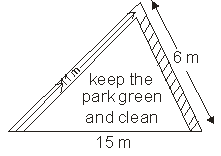Detailed Solution for Test: Introduction To Heron's Formula - Question 19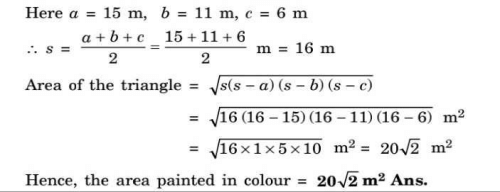Test: Introduction To Heron's Formula - Question 20

The area of a triangle whose sides are 13 cm, 14 cm and 15 cm is

Detailed Solution for Test: Introduction To Heron's Formula - Question 20

By using herons formula
We get s=13+14+15/2
S=21cm
Now area=√s(s-a) (s-b) (s-c)
√21(21-13)(21-14)(21-15)
√21(8)(7)(6)
√21(336)
√7056
84 cm² is the area.

## Mathematics (Maths) Class 9

88 videos|397 docs|109 tests
 Use Code STAYHOME200 and get INR 200 additional OFF Use Coupon Code
Information about Test: Introduction To Heron's Formula Page
In this test you can find the Exam questions for Test: Introduction To Heron's Formula solved & explained in the simplest way possible. Besides giving Questions and answers for Test: Introduction To Heron's Formula, EduRev gives you an ample number of Online tests for practice

## Mathematics (Maths) Class 9

88 videos|397 docs|109 tests